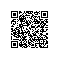# 技术交流笔记——SPRING IOC 原理

Class A {
B b;
C c;
public void service(){
Assert b!=null;
Assert c!= null;
....
}
}

Class B{
D d;
}

Class C{
E e;
F f;
}

Class D{}
Class E{}
Class F{}

class A{
public A(){
this.b=new B();
this.c=new C();
}
……
}
Class B{
public B(){
this.d=new D();
}
}
Class C{
public C(){
this.e=new E();
this.f=new F();
}
}

public static void main(String[] args){
A a=new A();
a.service();
}

Class A {

//B b;

D d;

C c;

public void service(){

Assert d!=null;

Assert c!= null;

....

}

}

class A{
public A(){
this.d=new D();
this.c=new C();
}
……
}
……

public static void main(String[] args){
A a=new A();
a.service();
}

Class A {

D d;

C c;

G g;

public void service(){

Assert d!=null;

Assert c!= null;

....

}

public void service_2(){

Assert g!= null;

……

}

}

class A{
public A(D _d, C _c){
this.d=_d;
this.c=_c;
}

public A(G _g){
this.g=_g;
}
……
}
……

public static void main(String[] args){
A a=new A(new D(),new C());
a.service();

A a_2=new A(new G());
a.service_2();
}

class A{
private static A a=new A(new D(),new C());
private static A a_2=new A(new G());

public static A getA(){
return a;
}

public static A getA_2(){
return a_2;
}

private A(D _d, C _c){
this.d=_d;
this.c=_c;
}

private A(G _g){
this.g=_g;
}
……
}
……

public static void main(String[] args){
A a=A.getA();
a.service();

A a_2=A.getA_2();
a.service_2();
}

Public abstract class Factory {

Public static Factory aFactory(){

Return new FactoryA ();

}

Public static Factory a2Factory(){

Return new FactoryA_2();

}

Create();

}

Public class FactoryA implements Factory {

@Override

Public A Create(){

return new A(new D(),new C());

}

}

Public class FactoryA_2 implements Factory {

@Override

Public A Create(){

return new A_2(new G());

}

}

……

public static void main(String[] args){

A a = Factory.aFactory().Create();

a.service();

A a_2 = Factory.a2Factory().Create();

a_2.service_2();

}

public Class Factory{

/**

* <p />

* String a = Factory.product(java.lang.String)，将返回一个””。等同于调用了 String a = new String();

*

* @param className 用户指定的类名。必须是全路径名，

* @return 用户指定的类的一个实例，或者是null

*/

public static Object product(String className){

}

}使用钉钉扫一扫加入圈子
+ 订阅